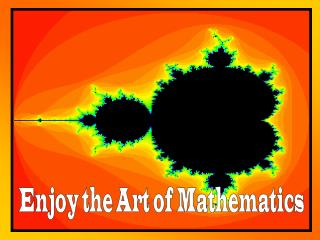DownloadDownload PresentationEnjoy the Art of Mathematics

# Enjoy the Art of Mathematics

Télécharger la présentation## Enjoy the Art of Mathematics

- - - - - - - - - - - - - - - - - - - - - - - - - - - E N D - - - - - - - - - - - - - - - - - - - - - - - - - - -
##### Presentation Transcript

1. Enjoy the Art of Mathematics

2. the Mandelbrot Set

3. Who Is Mandelbrot? • Benoit Mandelbrot • Mandelbrot was born in Poland in 1924. He studied mathematics in France under Gaston Julia and others. • Mandelbrot researched economic chaotic data for IBM. • In the 1970’s, he devised the geometry of "fractals.” • The name “fractal” comes from the Latin word fractus, meaning "to break."

4. What are fractals? • Fractals are shapes which have an overall structure that resembles each part of the structure’s smaller parts.

5. Do fractals occur naturally? • The term fractal can be used to describe the pattern in irregular shapes found in nature. • Plant growth patterns like broccoli • Waves and ripples in water • Mountain ranges • Snowflake edges • Cloud formations • Coastlines

6. What are fractals used for? • Fractals are used to describe and predict the changes in ecosystems. • Fractals are used to distinguish the strengths of metals. • Fractals are used to describe occurrences in meteorology and astronomy, like galaxy clusters. • Fractals are used to create computer images of landscapes for landscape design and science fiction films.

7. Fractals, like the Mandelbrot Set, are used in the classroom to encourage students to explore... • Patterns • Fraction division • and reduction • Scale and • magnification • Counting schemes • Coordinate systems • Integer arithmetic • Concept of Infinity

8. What is the Mandelbrot Set? • The Mandelbrot Set is a beautiful pattern of fractal geometry. • The Mandelbrot Set is the set of all Julia sets combined. Julia Set Mandelbrot Set

9. How is the Mandelbrot Set calculated? • Start with the quadratic function x2 + c, the simplest nonlinear function, where c is a constant. • Iterate (repeat) x2 + c by starting with a “seed” (any real or complex number used as a starting value). • Denote the seed by x0. Plug the seed x0 into the function x2 + c. • x1 = x02 + c.

10. Continue repeating this plugging-in operation, using the computation results from one equation as the input for the next equation. x2 = x12 + c, x3 = x22 + c, x4 = x32 + c, x5 = x42 + c, This repeated process is called the orbit of x0 .

11. The values from these equations are represented by colors . • Through computer imagery, the resulting beauty of these fractal shapes can be generated, such as the Mandelbrot Set.

12. The edges of the Mandelbrot Set can be magnified to reveal the amazing detail of the pattern. • There is infinite depth in one finite point.

13. Enjoy the art of mathematicsthrough fractal geometry.Explore the Mandelbrot Set.Create your own fractals on the web at: http://storm.shodor.org/eoe/mandy/index.html

14. References • Devlin, K. (1998). Life By the Numbers. New York: Wiley & Sons. • Suplee, C. (1999). Physics In the 20th Century. New York: Abrams. • http://archive.ncsa.uiuc.edu/Edu/Fractal/ Fractal_Home.html • http://library.thinkquest.org/3288.html • www.deepleaf.com/fractal/